# Text Analysis Using Turicreate

• Last Updated : 08 Dec, 2021

What is Text Analysis?
Text is a group of words or sentences.Text analysis is  analyzing the text and then extracting information with the help of text.Text data is one of  the biggest factor that can make a company big or small.For example

• On E-Commerce website people buy things .With Text Analysis the E-Commerce website can know what it’s customer likes and it through this data it can make it’s productivity higher.
• Using Text analysis and some Machine Learning Algorithm our Alexa Google Home mini works.  These two are based on Natural Language Processing.
• Using Text Analysis we can decide whether a E-mail is a Spam or a Non Spam.

Text analysis can be done using text mining.As the text “data” can be structured as well as unstructured.The text mining technique will help us in differentiating between them.

Now let’s do some text analysis using Turicreate.We will build a model that classifies that a message is a spam or ham for text analysis.Link for the dataset=https://www.kaggle.com/team-ai/spam-text-message-classification
Step 1: Import the Turicreate Library

## python3

 `import` `turicreate as tc`

## python3

 `data ``=` `tc.SFrame(``"data.csv"``)`

Step 3: We will explore the data first.

## python3

 `# It will print the first full rows of the data``data.head().`

`Output: `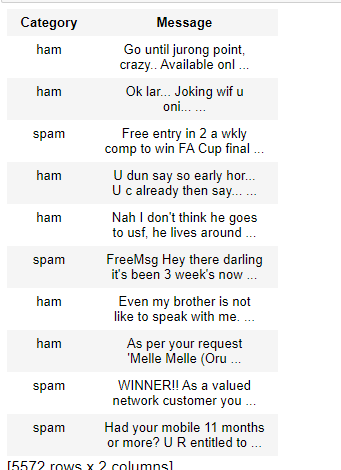dataset

Step 4:Now adding the word count in the data set.
This is because data has two things category and message. Adding the word count will help in model feature selection.

## python3

 `# Text analytics library has a count word function. ``# It will separately count the words for each row ``# of message column.``data[``'word_count'``]``=` `tc.text_analytics.count_words(data[``'Message'``])`` ` `# now we can see that the data has one more column if word_count.``data.head()`

Output: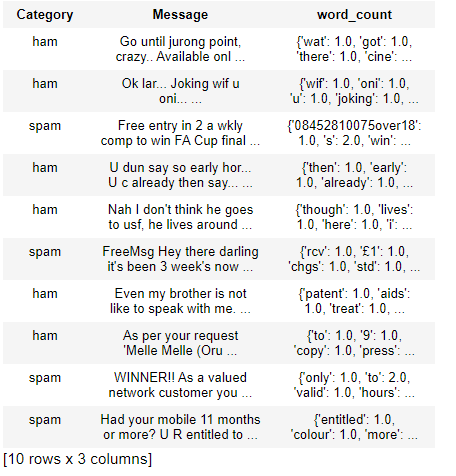Here One more row of word_count is added in the data set.

Step 5: To split the data into train and test set.

## python3

 `train_data, test_data ``=` `data.random_split(.``8``, seed ``=` `0``)`

Step 6: Now we will make a model for classifying the spam and ham.

## python3

 `# We will use our feature as word count and ``# our target "category is to find out spam or ham.`` ` `model ``=` `tc.logistic_classifier.create(``    ``train_data, target ``=``'Category'``, ``    ``features ``=``[``'word_count'``], ``    ``validation_set ``=` `test_data)`

Step 7: Now we will check accuracy of our model.

## python3

 `model.evaluate(test_data)`

Output: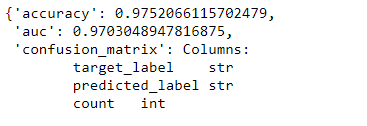The accuracy is 0.975 that means 97.5%.Step 8: We can predict manually by checking from our test data that it is giving right answer or not.

Code:

## python3

 `test_data.head()``# We will select the first one that is spam ``# and select that is spam or not.`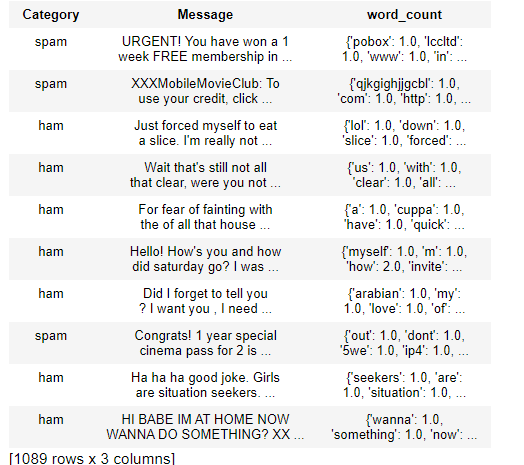Step 9: Predicting the test data.

## python3

 `model.predict(test_data[``1``])`

Output: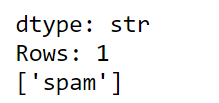The result is spam hence the model is predicting it right.

My Personal Notes arrow_drop_up